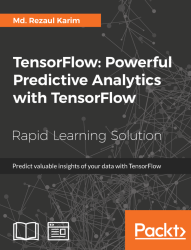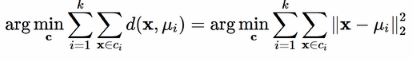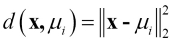•#### TensorFlow: Powerful Predictive Analytics with TensorFlow#### Overview of this book

Predictive analytics discovers hidden patterns from structured and unstructured data for automated decision making in business intelligence. Predictive decisions are becoming a huge trend worldwide, catering to wide industry sectors by predicting which decisions are more likely to give maximum results. TensorFlow, Google’s brainchild, is immensely popular and extensively used for predictive analysis. This book is a quick learning guide on all the three types of machine learning, that is, supervised, unsupervised, and reinforcement learning with TensorFlow. This book will teach you predictive analytics for high-dimensional and sequence data. In particular, you will learn the linear regression model for regression analysis. You will also learn how to use regression for predicting continuous values. You will learn supervised learning algorithms for predictive analytics. You will explore unsupervised learning and clustering using K-meansYou will then learn how to predict neighborhoods using K-means, and then, see another example of clustering audio clips based on their audio features. This book is ideal for developers, data analysts, machine learning practitioners, and deep learning enthusiasts who want to build powerful, robust, and accurate predictive models with the power of TensorFlow. This book is embedded with useful assessments that will help you revise the concepts you have learned in this book. This book is repurposed for this specific learning experience from material from Packt's Predictive Analytics with TensorFlow by Md. Rezaul Karim.
TensorFlow: Powerful Predictive Analytics with TensorFlowCreditsPrefaceFree Chapter
From Data to Decisions – Getting Started with TensorFlowPutting Data in Place – Supervised Learning for Predictive AnalyticsClustering Your Data – Unsupervised Learning for Predictive AnalyticsUsing Reinforcement Learning for Predictive Analytics## Using K-means for Predictive Analytics

K-means is a clustering algorithm that tries to cluster related data points together. However, we should know its working principle and mathematical operations.

### How K-means Works

Suppose we have n data points, xi, i = 1...n, that need to be partitioned into k clusters. Now that the target here is to assign a cluster to each data point, K-means aims to find the positions, μi, i=1...k, of the clusters that minimize the distance from the data points to the cluster. Mathematically, the K-means algorithm tries to achieve the goal by solving an equation that is an optimization problem:In the previous equation, ci is a set of data points, which when assigned to cluster i andis the Euclidean distance to be calculated (we will explain why we should use this distance measurement shortly). Therefore, we can see that the overall clustering operation using K-means is not a trivial one, but a NP-hard optimization problem. This also means that the K-means algorithm...